# Complex Transformations with Clicks in Tableau Prep: Introducing Multi-row Calculations

Discover how to use multi-row calculations to compute table calculations while preparing your data using clicks, not code or complex calculations!

Starting in Tableau Prep 2023.2, you can now use multi-row calculations to compute table calculations while preparing your data. Using clicks, not code or complex calculations, we’ve added visual experiences that make it easier to calculate difference from, percent difference from, and moving calculations. For you power users out there, you can now write LOOKUP calculations in the expression editor in Tableau Prep.

While multi-row and table calculations can produce the same results, they do so in fundamentally different ways. Three key benefits of multi-row calculations are:

1. Improved dashboard performance: Reduce view load times for large datasets by computing multi-row calculations at the physical layer.
2. Simplified interactivity: Workbook filters won’t change how your table calculation is computed.
3. Increased usability: Use multi-row calculations in subsequent calculations.

In Tableau Desktop, table calculations are powerful tools that enable analysis on top of the measures and dimensions in your analytics view. Table calculation flexibility while exploring data is liberating, but productionalizing content containing them can be frustrating. Table calculations are computed after the query returns, so they only operate on the values in the query results. Table calculations can also slow down workbook load times when used on large data sets, limit additional visualization interactivity, and impact data accuracy if not applied correctly.

Multi-row calculations in Tableau Prep give you the choice to push these table calculations down to the physical data layer for faster dashboard load times with large data sets. It also allows you to filter your measures and dimensions without having to consider the impact it may have on how the underlying table calculation is computed. Today in Tableau Desktop, any computation done on a table calculation result must be another table calculation, so doing these calculations in Tableau Prep allows you to add subsequent calculations that aren’t limited to table calculations.

Plus, adding this field to the dataset means it’s reusable and others in your organization can leverage it. Depending on the level of interactivity and usability you need, consider using multi-row calculations instead of table calculations, or a combination of both.

Let’s walk through how to use multi-row calcs in Tableau Prep to wrangle sales data and ultimately create a dashboard that tracks moving averages across different regions over time.

## Tracking Moving Averages of Sales across Regions over Time

We’ll be using the Superstore sample flow that comes with your Tableau Prep 2023.2 download in this example. Imagine you’re an online store regional sales manager. Your goal is to track the three month moving average of sales per region. To do this, you’ll use a multi-row calculation in Tableau Prep to ensure your sales managers can filter on month without having to worry about impacting calculation results.

Open the Superstore flow, open the Roll Up Sales Aggregation step, and add [Order Date] to Grouped Fields. Next, convert Order Date to Month Number using the More options menu. Lastly, rename the field to [Order Month]. This will generate the total monthly sales per region over the years.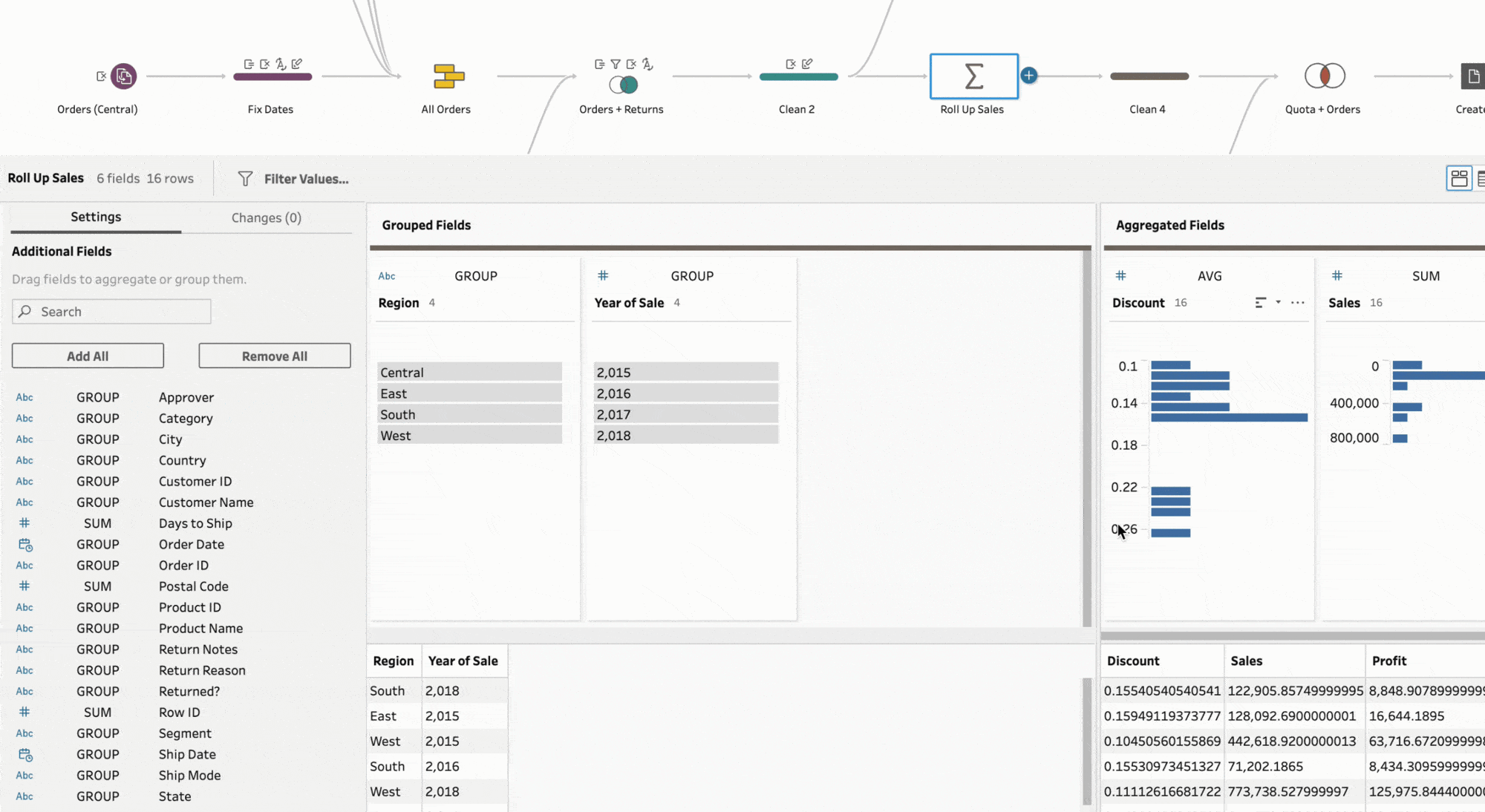Next, create a new Clean step and locate the [Sales], [Region], [Order Month], and [Year of Sale] fields. Select the [Sales] field, and select Moving Calculation from the More options menu.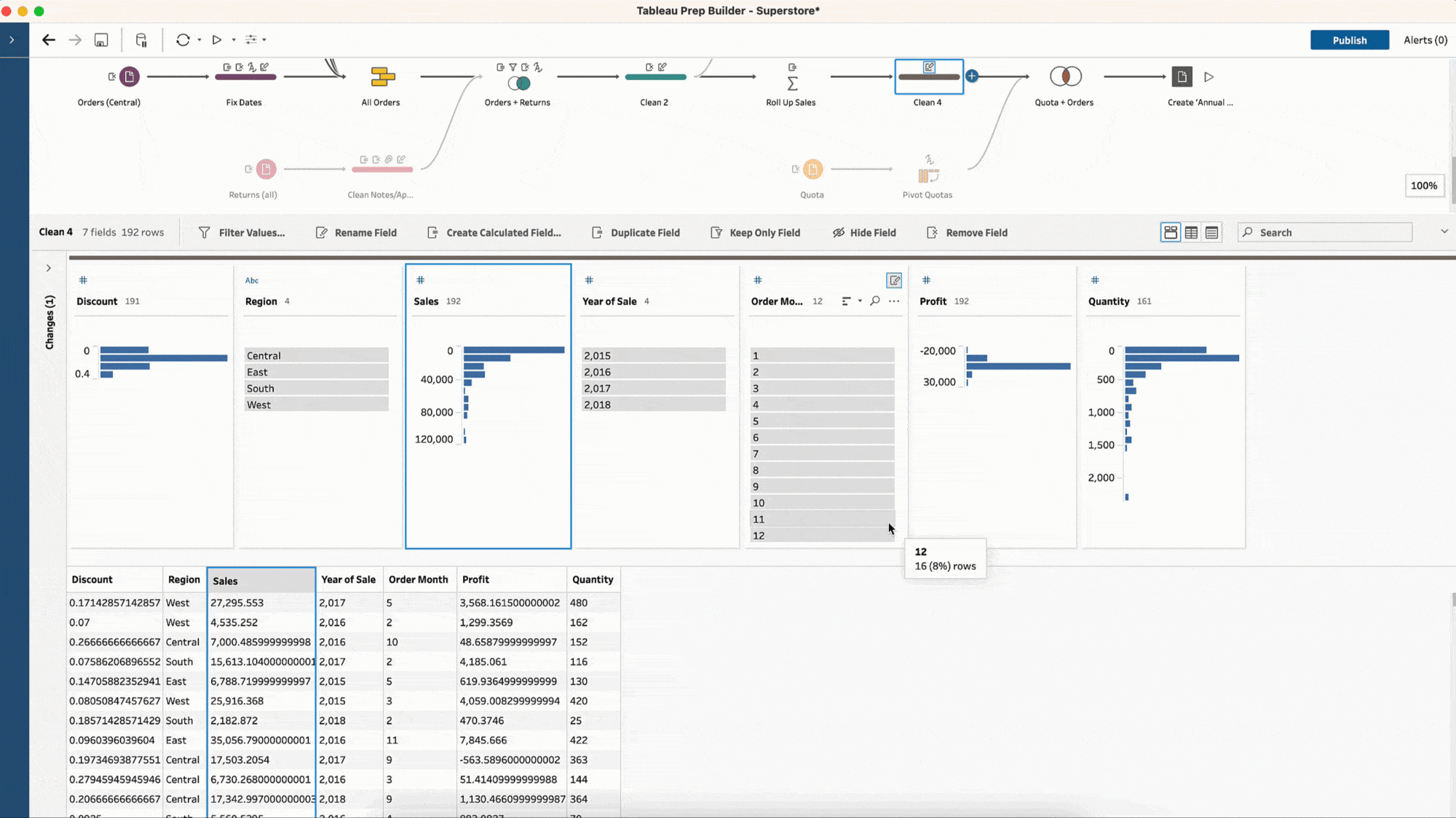You are computing the average of [Sales], but want to do so individually for each region, so you will group by [Region]. Next, order by the [Year of Sale] and [Order Month] fields in ascending order and change the result type to a moving AVG instead of a moving SUM.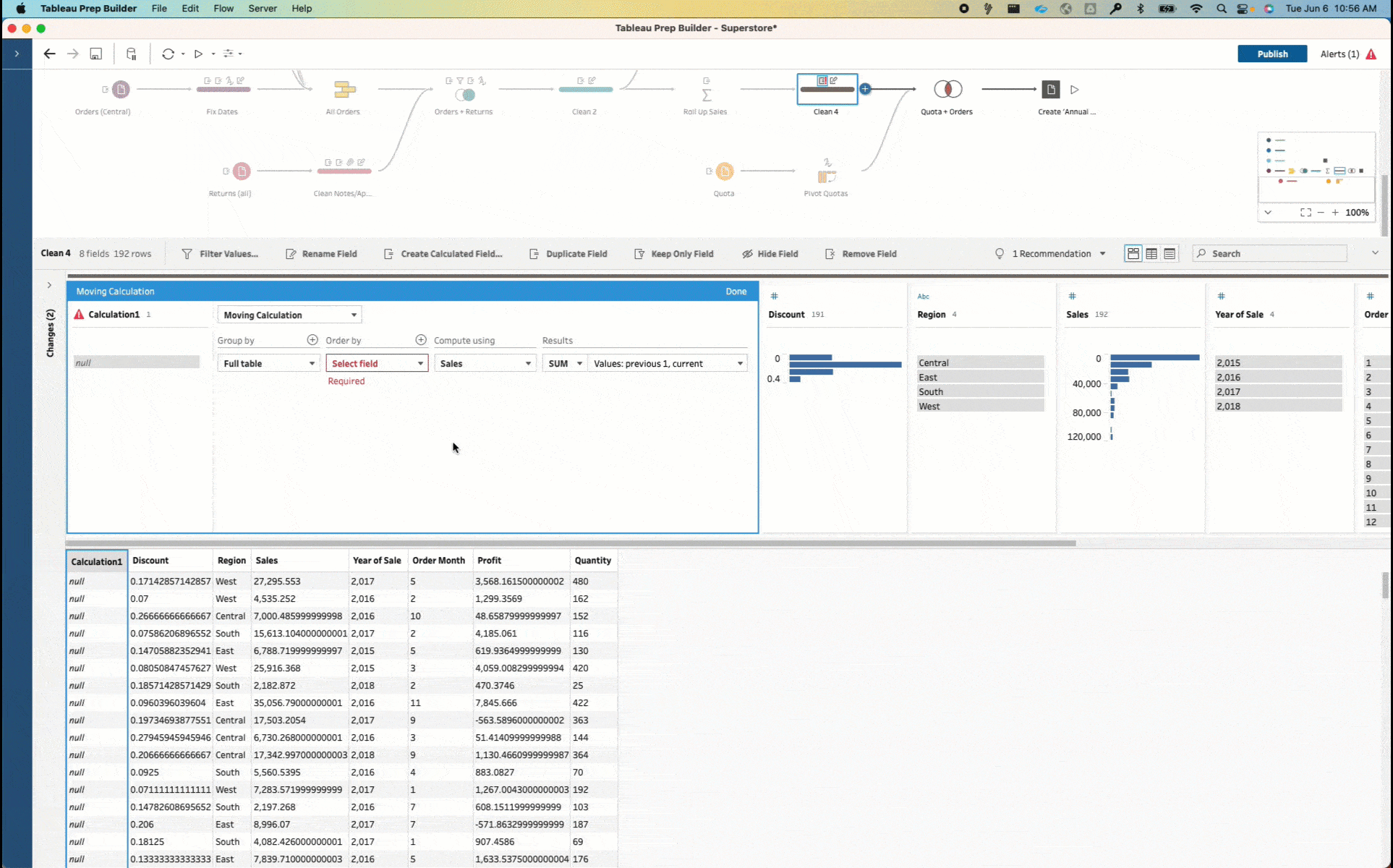Notice the resulting values in the visual calculation editor are interactive. You can see annotations that help you understand how the calculation has been created. You can also change the number of previous or next values depending on the type of moving average you want. We want this moving average to be across the previous two months and the current, so you will increase the number of previous values to two.

Note that when working with date data, you might have some gaps in your dates; this is a common scenario, so consider scaffolding your date field with a New Rows step depending on your analysis goals.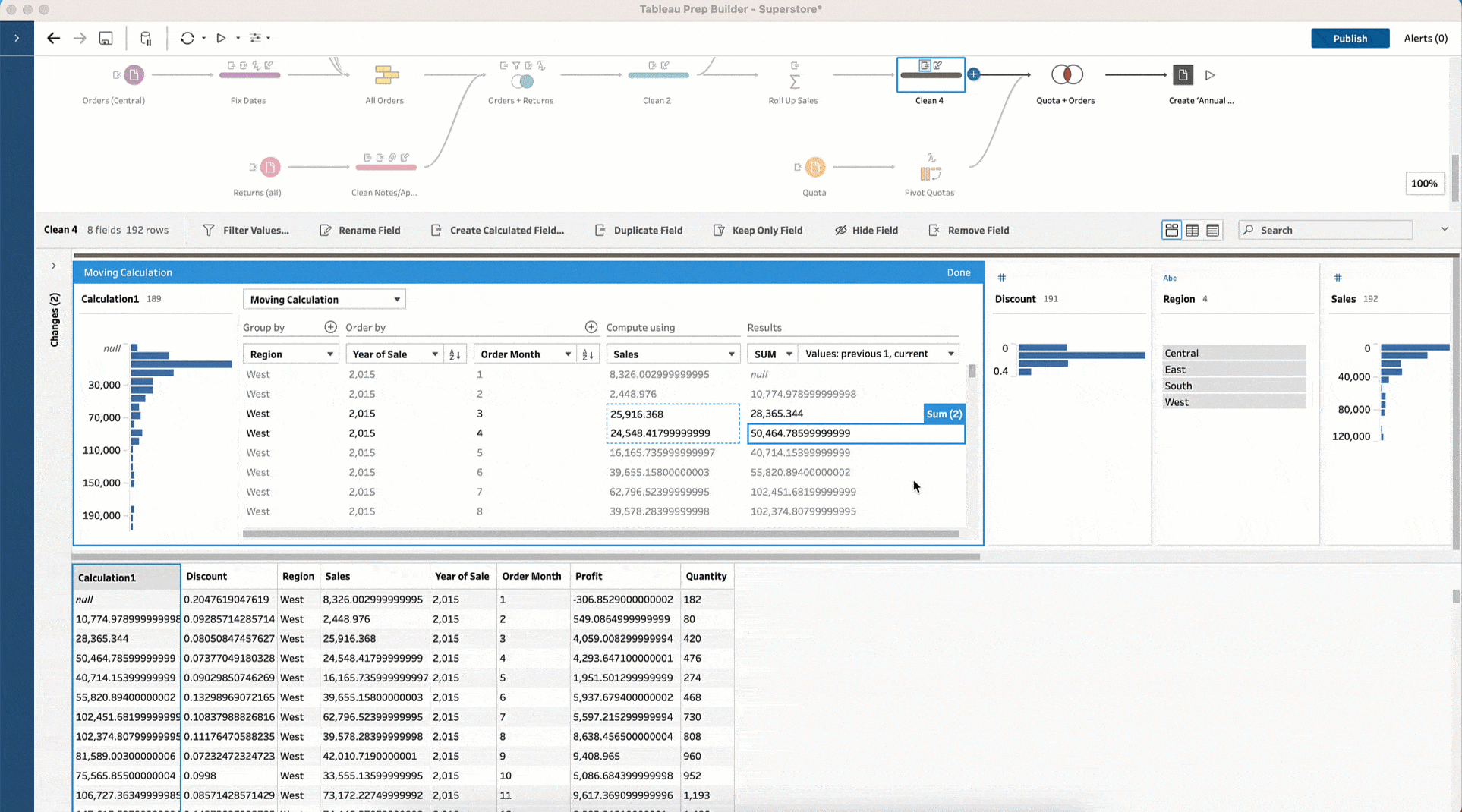The last step is to clearly name the calculated field, describing how the calculation has been created — in this case “3 Month Moving Average of Sales for each Region”. Proper field descriptions and documentation ensure that others in your organization can understand and use the calculated field correctly.

Once you’ve configured the calculation, you can run your Tableau Prep flow to generate a published data source and create a downstream workbook. Now you and your viewers can filter on date on top of this field in the workbook without it changing the results.

With Tableau Data Management, you can schedule this Tableau Prep flow to update monthly with the freshest data — ensuring your dashboards will have fast, up-to-date loading information so you can easily, accurately interact with your table calculations.

Let’s take a look at how this approach impacted the West region in our Superstore data: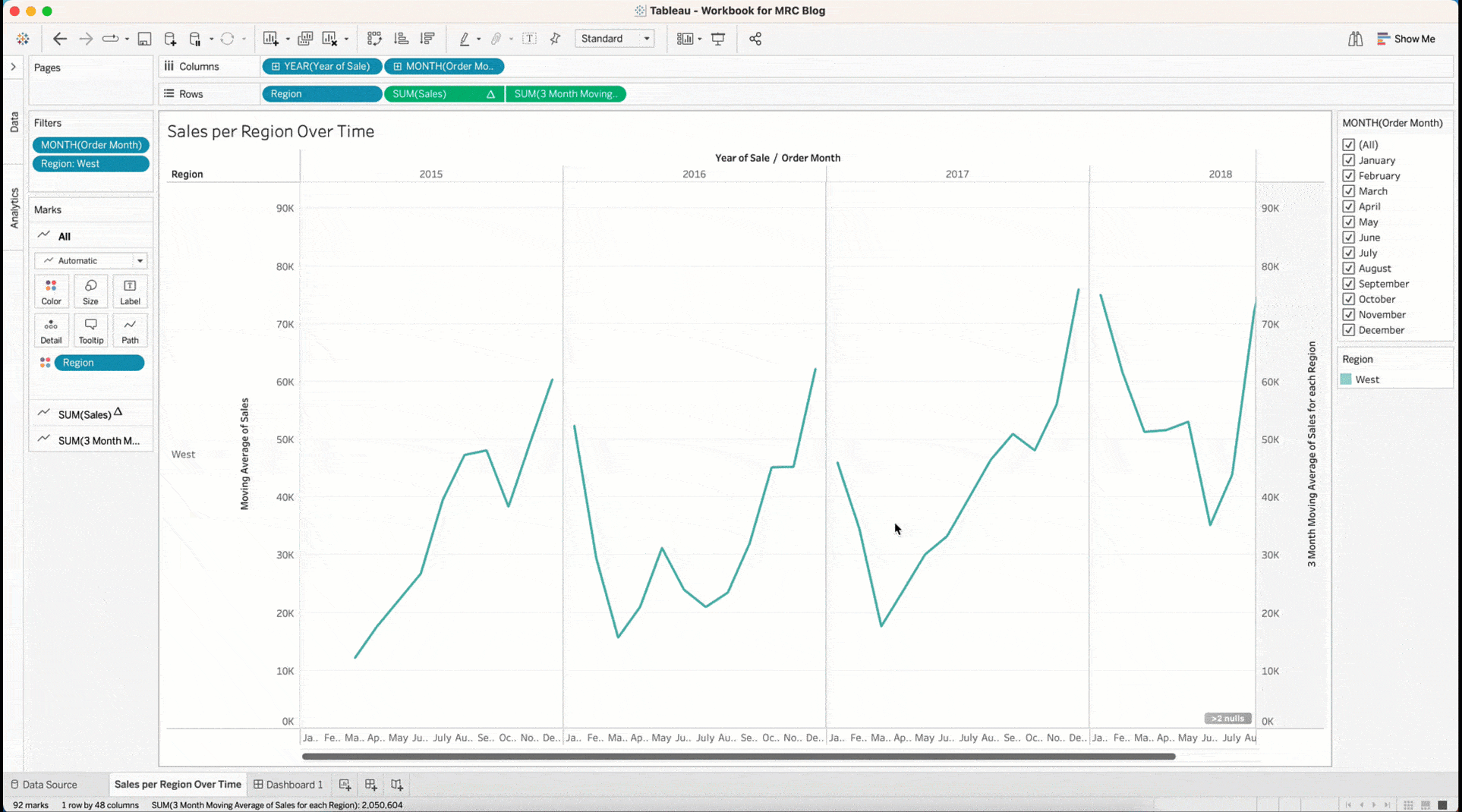This Tableau workbook compares two ways of calculating the moving monthly average — computing with multi-row calculations in Tableau Prep (opaque line) and computing with table calculations in Tableau Desktop (transparent line). When filtering on month, notice how the  moving average calculation computed in Prep does not change based on the months in the view, whereas the moving average in Desktop does, which could be confusing to the viewers interacting with your dashboard.

## Get hands on with multi-row calculations in Tableau Prep

Multi-row calculations can improve dashboard performance, simplify interactivity and increase the usability of your fields, and we can’t wait to learn about all the ways you will take advantage of these benefits!